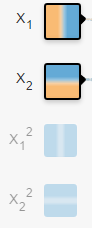A-A+

# 如何简单形象又有趣地讲解神经网络是什么？

2016年10月19日 阅读 4,083 次

1、神经网络为什么可以用于识别

2、神经网络变深后我们获得了什么

3、“过深”的网络的效果又变差的原因

4、“深浅”会影响神经网络表现的背后原因

5、RNN、CNN以及各种不同网络结构的共性是什么

6、设计神经网络的本质是什么

## 目录

• 一基本变换层
• 二理解视角
• 数学视角线性可分
• 物理视角物质组成
• 三神经网络的训练
• 如何训练
• 梯度下降的问题
• 1局部极小值
• 2梯度的计算
• 基本流程图
• 四深层的思考真的只有这些原因吗

## 一、基本变换：层神经网络是由一层一层构建的，那么每层究竟在做什么？

• 数学式子：$\vec{y}= a(W\cdot\vec{x} + {b})$，其中$\vec{x}$是输入向量，$\vec{y}$是输出向量，$\vec{b}$是偏移向量，$W$是权重矩阵，$a()$是激活函数。每一层仅仅是把输入$\vec x$经过如此简单的操作得到$\vec y$
• 数学理解：通过如下5种对输入空间（输入向量的集合）的操作，完成 输入空间 ——> 输出空间 的变换 (矩阵的行空间到列空间)。
注：用“空间”二字的原因是被分类的并不是单个事物，而是一类事物。空间是指这类事物所有个体的集合。

• 1. 升维/降维
• 2. 放大/缩小
• 3. 旋转
• 4. 平移
• 5. “弯曲”
这5种操作中，1,2,3的操作由$W\cdot\vec{x}$完成，4的操作是由$+\vec{b}$完成，5的操作则是由$a()$来实现。 (此处有动态图5种空间操作，帮助理解)

• 物理理解：对 $W\cdot\vec{x}$ 的理解就是通过组合形成新物质。$a()$又符合了我们所处的世界都是非线性的特点。
• 情景：$\vec{x}$是二维向量，维度是碳原子和氧原子的数量$[C;O]$，数值且定为$[1;1]$，若确定$\vec{y}$是三维向量，就会形成如下网络的形状 (神经网络的每个节点表示一个维度)。通过改变权重的值，可以获得若干个不同物质。右侧的节点数决定了想要获得多少种不同的新物质。（矩阵的行数）
•• 1.如果权重W的数值如（1），那么网络的输出y 就会是三个新物质，[二氧化碳，臭氧，一氧化碳]。
\begin{equation}\begin{bmatrix}CO_{2}\\O_{3}\\CO\end{bmatrix}=\begin{bmatrix}1&2\\0&3\\1&1\end{bmatrix}\cdot\begin{bmatrix}C\\O\end{bmatrix}\end{equation}
• 2.也可以减少右侧的一个节点，并改变权重W至（2），那么输出$\vec{y}$ 就会是两个新物质，$[ O_{0.3};CO_{1.5}]$
\begin{equation}\begin{bmatrix}CO_{2}\\O_{3}\\CO\end{bmatrix}=\begin{bmatrix}1&2\\0&3\\1&1\end{bmatrix}\cdot \begin{bmatrix}C\\O\end{bmatrix}\end{equation}
• 3.如果希望通过层网络能够从[C, O]空间转变到$[CO_{2};O_{3};CO]$空间的话，那么网络的学习过程就是将W的数值变成尽可能接近(1)的过程 。如果再加一层，就是通过组合$[CO_{2};O_{3};CO]$这三种基础物质，形成若干更高层的物质。
• 4.重要的是这种组合思想，组合成的东西在神经网络中并不需要有物理意义。

## 二、理解视角：现在我们知道了每一层的行为，但这种行为又是如何完成识别任务的呢？

• 一维情景：以分类为例，当要分类正数、负数、零，三类的时候，一维空间的直线可以找到两个超平面（比当前空间低一维的子空间。当前空间是直线的话，超平面就是点）分割这三类。但面对像分类奇数和偶数无法找到可以区分它们的点的时候，我们借助 x % 2（取余）的转变，把x变换到另一个空间下来比较，从而分割。• 二维情景：平面的四个象限也是线性可分。但下图的红蓝两条线就无法找到一超平面去分割。神经网络的解决方法依旧是转换到另外一个空间下，用的是所说的5种空间变换操作。比如下图就是经过放大、平移、旋转、扭曲原二维空间后，在三维空间下就可以成功找到一个超平面分割红蓝两线 (同SVM的思路一样)。上面是一层神经网络可以做到的，如果把$\vec{y}$ 当做新的输入再次用这5种操作进行第二遍空间变换的话，网络也就变为了二层。最终输出是$\vec{y}= a_{2}(W_{2}\cdot(a_{1}(W_{1}\cdot\vec{x} + {b}_{1})) + {b}_{2})$
设想网络拥有很多层时，对原始输入空间的“扭曲力”会大幅增加，如下图，最终我们可以轻松找到一个超平面分割空间。当然也有如下图失败的时候，关键在于“如何扭曲空间”。所谓监督学习就是给予神经网络网络大量的训练例子，让网络从训练例子中学会如何变换空间。每一层的权重W就控制着如何变换空间，我们最终需要的也就是训练好的神经网络的所有层的权重矩阵。。这里有非常棒的可视化空间变换demo，一定要打开尝试并感受这种扭曲过程。更多内容请看Neural Networks, Manifolds, and Topology• 类比：回想上文由碳氧原子通过不同组合形成若干分子的例子。从分子层面继续迭代这种组合思想，可以形成DNA，细胞，组织，器官，最终可以形成一个完整的人。继续迭代还会有家庭，公司，国家等。这种现象在身边随处可见。并且原子的内部结构与太阳系又惊人的相似。不同层级之间都是以类似的几种规则再不断形成新物质。你也可能听过分形学这三个字。可通过观看从1米到150亿光年来感受自然界这种层级现象的普遍性。• 人脸识别情景：我们可以模拟这种思想并应用在画面识别上。由像素组成菱角再组成五官最后到不同的人脸。每一层代表不同的不同的物质层面 (如分子层)。而每层的W存储着如何组合上一层的物质从而形成新物质。
如果我们完全掌握一架飞机是如何从分子开始一层一层形成的，拿到一堆分子后，我们就可以判断他们是否可以以此形成方式，形成一架飞机。
附：Tensorflow playground展示了数据是如何“流动”的。## 三、神经网络的训练知道了神经网络的学习过程就是学习控制着空间变换方式（物质组成方式）的权重矩阵后，接下来的问题就是如何学习每一层的权重矩阵W。

1、局部极小值梯度下降寻找的是loss function的局部极小值，而我们想要全局最小值。如下图所示，我们希望loss值可以降低到右侧深蓝色的最低点，但loss有可能“卡”在左侧的局部极小值中。• 调节步伐：调节学习速率，使每一次的更新“步伐”不同。常用方法有：
• 动量（Momentum）：不仅仅考虑当前样本所计算的梯度；Nesterov动量（Nesterov Momentum）：Momentum的改进
• 优化起点：合理初始化权重（weights initialization）、预训练网络（pre-train），使网络获得一个较好的“起始点”，如最右侧的起始点就比最左侧的起始点要好。常用方法有：高斯分布初始权重（Gaussian distribution）、均匀分布初始权重（Uniform distribution）、Glorot 初始权重、He初始权、稀疏矩阵初始权重（sparse matrix）

2、梯度的计算机器学习所处理的数据都是高维数据，该如何快速计算梯度、而不是以年来计算。• 收集训练集（train data）：也就是同时有input以及对应label的数据。每个数据叫做训练样本（sample）。label也叫target，也是机器学习中最贵的部分。上图表示的是我的数据库。假设input本别是x的维度是39，label的维度是48。
• 设计网络结构（architecture）：确定层数、每一隐藏层的节点数和激活函数，以及输出层的激活函数和损失函数。上图用的是两层隐藏层（最后一层是输出层）。隐藏层所用激活函数a( )是ReLu，输出层的激活函数是线性linear（也可看成是没有激活函数）。隐藏层都是1000节点。损失函数L( )是用于比较距离MSE：mean((output - target)^2)。MSE越小表示预测效果越好。训练过程就是不断减小MSE的过程。到此所有数据的维度都已确定：
• 训练数据：$input \in R^{39} ;label \in R^{48}$
• 权重矩阵：$W_{h1}\in R^{1000x39};W_{h2}\in R^{1000x1000} ;W_{o}\in R^{48x1000}$
• 偏移向量：$b_{h1}\in R^{1000};b_{h2}\in R^{1000} ;b_{o}\in R^{48}$
• 网络输出：$output \in R^{48}$
• 数据预处理（preprocessing）：将所有样本的input和label处理成能够使用神经网络的数据，label的值域符合激活函数的值域。并简单优化数据以便让训练易于收敛。比如中心化（mean abstraction）、归一化（normlization）、主成分分析（PCA）、白化（whitening）。假设上图的input和output全都经过了中心化和归一化。
• 权重初始化（weights initialization）：$W_{h1},W_{h2},W_{0}$在训练前不能为空，要初始化才能够计算loss从而来降低。$W_{h1},W_{h2},W_{0}$初始化决定了loss在loss function中从哪个点开始作为起点训练网络。上图用均匀分布初始权重（Uniform distribution）。
• 正向传递：，算当前网络的预测值$output =linear (W_{o} \cdot Relu(W_{h2}\cdot Relu(W_{h1}\cdot input+b_{h1})+b_{h2}) +b_{o})$
• 计算loss：$loss = mean((output - target)^2)$• 更新权重：这里用最简单的方法来更新，即所有参数都 $W = W - learningrate * gradient$
• 预测新值：训练过所有样本后，打乱样本顺序再次训练若干次。训练完毕后，当再来新的数据input，就可以利用训练的网络来预测了。这时的output就是效果很好的预测值了。下图是一张实际值和预测值的三组对比图。输出数据是48维，这里只取1个维度来画图。蓝色的是实际值，绿色的是实际值。最上方的是训练数据的对比图，而下方的两行是神经网络模型从未见过的数据预测对比图。（不过这里用的是RNN，主要是为了让大家感受一下效果）• 数据：在二维平面内，若干点被标记成了两种颜色。黄色，蓝色，表示想要区分的两类。你可以把平面内的任意点标记成任意颜色。网页给你提供了4种规律。神经网络会根据你给的数据训练，再分类相同规律的点。• 输入：在二维平面内，你想给网络多少关于“点”的信息。从颜色就可以看出来，$x_{1}$左边是负，$x_{1}$右边是正，表示此点的横坐标值。同理，$x_{2}$表示此点的纵坐标值。$x_{1}^{2}$是关于横坐标值的“抛物线”信息。你也可以给更多关于这个点的信息。给的越多，越容易被分开。• 连接线：表示权重，蓝色表示用神经元的原始输出，黄色表示用负输出。深浅表示权重的绝对值大小。鼠标放在线上可以看到具体值。也可以更改。在（1）中，当把$x_{2}$输出的一个权重改为-1时，$x_{2}$的形状直接倒置了。不过还需要考虑激活函数。（1）中用的是linear。在（2）中，当换成sigmoid时，你会发现没有黄色区域了。因为sigmoid的值域是(0,1)

• 输出：黄色背景颜色都被归为黄点类，蓝色背景颜色都被归为蓝点类。深浅表示可能性的强弱。上图中所有在黄色背景颜色的点都会被分类为“黄点“，同理，蓝色区域被分成蓝点。在上面的分类分布图中你可以看到每一层通过上一层信息的组合所形成的。权重（那些连接线）控制了“如何组合”。神经网络的学习也就是从数据中学习那些权重。Tensorflow playground所表现出来的现象就是“在我文章里所写的“物质组成思想”，这也是为什么我把Tensorflow playground放在了那一部分。

5种空间变换的理解：Tensorflow playground下没有体现5种空间变换的理解。需要打开这个网站尝试：ConvNetJS demo: Classify toy 2D data## 四、“深层”的思考：真的只有这些原因吗？文章的最后稍微提一下深层神经网络。深层神经网络就是拥有更多层数的神经网络。